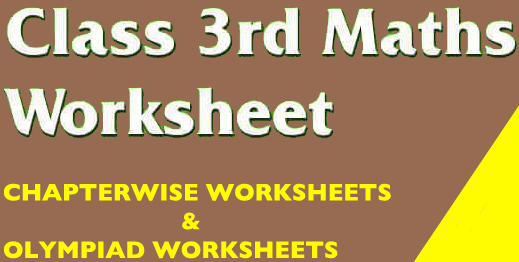);

# CBSE Sample Papers, Worksheets

Schools, Students & Parents – Connected

## Get Maths Worksheets for Grade 3 in Printable PDFEvery School those come under the affiliation of Central Board of Schools Education ( CBSE) have one thing in common , and that is they follow the NCERT curriculum and books. The National Council of Education Research and Training ( NCERT ) always focus on designing the curriculum in a planned manner so that students would remain engaged with learning and get acquainted with a variety of important topics.In class 3, the maths curriculum is drafted and designed in an engaging and interesting way. Students are introduced and exposed to various important maths topics in the form of various illustrations and practical examples.The Syllabus for Class 3 Maths covers topics like addition, subtraction basic geometry, Fun with numbers, Indian Currency, Time and Clock and many more

# CBSE Class 3 Maths Worksheets

Addition Sums for Class 3 Practice Worksheet

Subtraction Sum for Class 3 Practice Worksheets

Multiplication Sums for Class 3 Practice Worksheets

Maths Patterns for Class 3 Worksheets

CBSE Class 3 Maths Worksheets – Data Handling

Division Sums for Class 3 Practice Worksheet

CBSE Class 3 Maths Worksheets – Geometry

Fun with Numbers Class 3 Worksheet

CBSE Class 3 Maths Worksheets – Give & Take

CBSE Class 3 Maths Worksheets – Litres and Millilitres

Measurements Worksheet for Grade 3 CBSE

Mental Maths Worksheets for Class 3

CBSE Class 3 Maths Worksheets – Patterns

CBSE Class 3 Maths Worksheets – Rupees & Paisa Currency

CBSE Class 3 Maths Worksheets – Shapes and Designs

CBSE Class 3 Maths Worksheets – Time and Clock

CBSE Class 3 Maths Worksheets – Understanding Weights

#### Maths Olympiad for Class 3 Worksheets | International Mathematics Olympiad (IMO)

Maths Olympiad for Class 3 Division Worksheets

CBSE Class 3 Maths Worksheets ( Fraction ) – Maths Olympiad Preparation

Maths Olympiad for Class 3 Multiplication Worksheets

Class 3 Maths Worksheets ( Place Value ) Olympiad

Maths Olympiad for Class 3 Subtraction Worksheets

CBSE Class 3 Maths Worksheets ( Mental Ability ) – Maths Olympiad

TERM-WISE SYLLABUS
Session – 2019-20
Class 3 Subject: Mathematics

1. Work with three digit numbers:
1.1 Reads and writes numbers upto 1000.
1.2 Compares numbers up to 1000.
1.3 Solves simple daily life problems using addition and subtraction of three digit numbers.
1.4 Constructs and uses the multiplication facts (table) of 2, 3, 4, 5 and 10 in daily life situations.
1.5 Analyzes and applies an appropriate number operation in the situation / context.
1.6 Explains the meaning of division facts by equal grouping / sharing and finds it by repeated subtraction.
For example 12, 3 as number of groups of 3 to make 12 and finds it as 4 by repeatedly subtracting 3 from 12.
1.7 Can split numbers into hundreds, tens and ones. For e.g. 354 is 300 and 50 and 4.
1.8 Adds and subtracts small amounts of money with or without regrouping.
1.9 Makes rate charts and simple bills.
1.10 Can visualise a situation given in a word problem and choose the appropriate operation.
2. Acquires understanding about 2D shapes
2.1 Identifies and makes 2D- shapes by paper folding, paper cutting on the dot grid, using straight lines etc.
2.2 Describes 2D shapes by the number of sides, corners and diagonals. For example, the shape of the book
cover has 4 sides, 4 corners and two fills a given region leaving no gaps using a tile of a given shape.
3. Estimates and measures length and distance using standard units like centimeters or meters & identifies relationships.
4. Weights objects using standard units – grams & kilograms using simple balance.
5. Compares the capacity of different containers in terms of non-standard units.
6. Adds & subtracts measure involving grams & kilograms in life situations.
7. Identifies a particular day and date on a calendar.
8. Reads the time correctly to the hour using a clock / watch.
9. Extends pattern in simple shapes and numbers.
10. Acquires understanding about data handling.
10.1 Records data using tally marks, represents pictorially and draws conclusions.